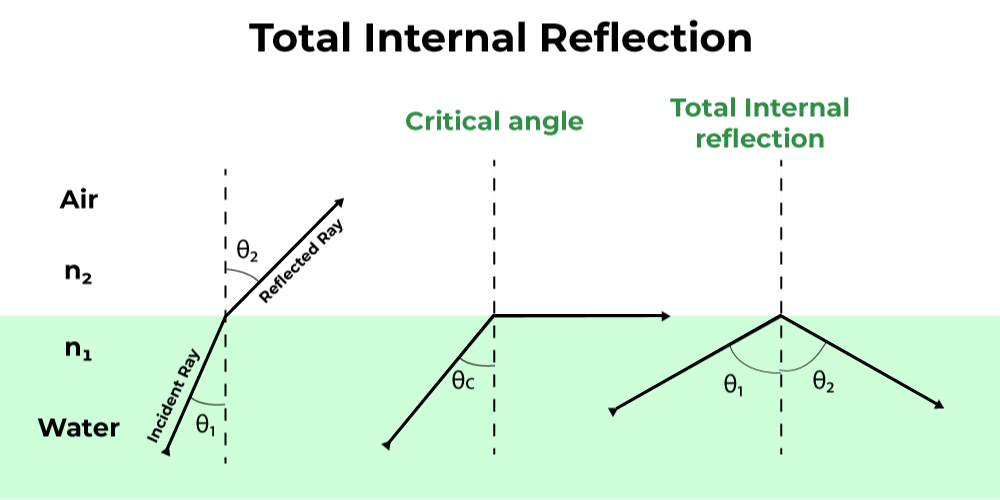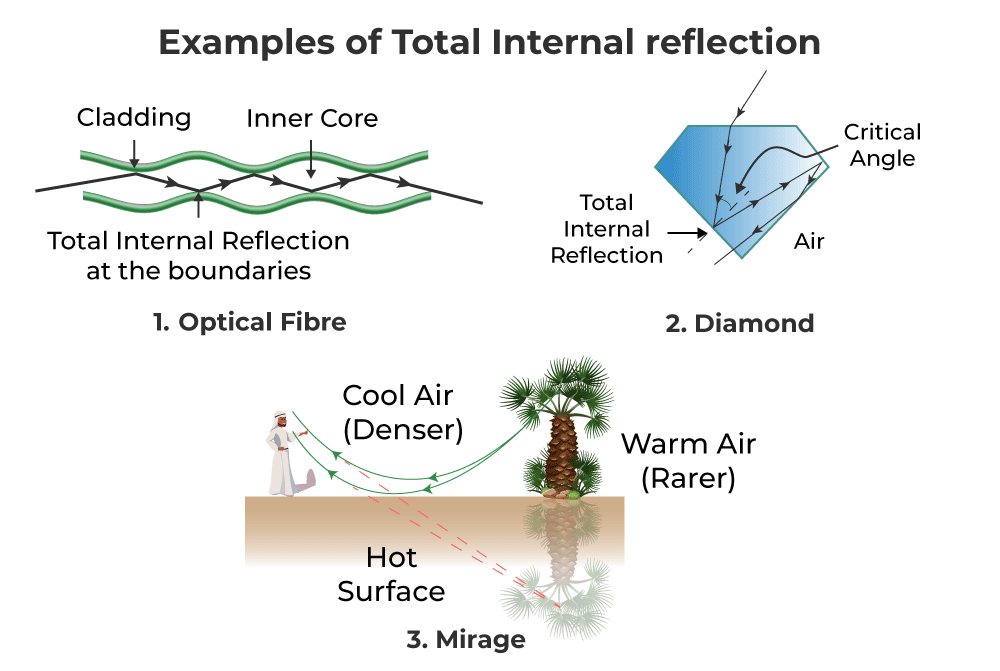GFG App
Open AppBrowser
Continue

# Total Internal Reflection

In Physics, total internal reflection is the complete reflection of a light ray within the medium (air, water glass, etc). For example, the total internal reflection of rays of light takes place in a Diamond. Since Dimond has multiple reflecting surfaces through which the Total internal reflection takes place. The total internal reflection occurs when light travels from a denser medium to a less dense medium and the angle of incidence must be greater than the critical angle. The phenomenon of total internal reflection has a wide application in optical devices such as telescopes, binoculars, periscopes, etc. Read more about the concepts of Total Internal Reflection like Total Internal Reflection of Light, definition, critical angle, and total internal reflection, examples, and FAQs, here in this article!

## What is Total Internal Reflection?

The complete reflection of a light ray at the boundary of two media when the beam is in the medium with a higher refractive index is known as total internal reflection.

This is due to the fact that when there is water in the glass, light from the coin travels through the glass at a certain angle to our sight. Further, when water is added to the glass, the light from the coil strikes the interior of the glass at a higher angle than the critical angle. The glass inside reflects 100% of the light. Internal reflection is what it is.

## Conditions for Total Internal Reflection

When light beams go from a more optically dense material to a less optically dense medium, this phenomenon happens. There are two situations in which total internal reflection occurs:

• When the light is approaching the less dense medium from the more dense medium.
• If the incidence angle is larger than the critical angle.

## Total Internal Reflection Formula

Consider the case below. A beam of light travels from a watery medium to one of air. The light ray will be refracted at the point where the two mediums meet. The refracted light beam bends away from the normal as it passes from a medium with a higher refractive index to one with a lower refractive index.

• The incident beam of light is refracted in such a way that it travels over the water’s surface at a certain angle of incidence.
• The critical angle is the angle of incidence at which something happens. The refraction angle is 90° here.
• The incident ray is reflected back to the medium when the angle of incidence is larger than the critical angle. This event is referred to as total internal reflection.The formula for total internal reflection is stated as:

n1 ⁄ n2 = sin θ1 ⁄ sin θ2

where,

• θ1 is the angle of incidence,
• θ2 is the angle of refraction,
• n1 is the denser medium, &
• n2 is the rarer medium.

### Critical Angle

Critical angle is defined as the largest angle of incidence for which refraction of light inside the medium still can be possible. Therefore, the angle of incidence, when the angle of refraction is 90 degrees and the ray which is refracting to total internal refraction is called the critical angle.

Mathematically, the critical angle for a total internal reflection is defined as,

θc = sin−1(n2 ⁄ n1)

where,

• θc is the critical angle.
• n1 is the denser medium, &
• n2 is the rarer medium.

## Total Internal Reflection Examples### Total Internal Reflection in Optical Fiber

Because the angle generated by the incoming beam is higher than the critical angle, total internal reflection occurs when it strikes the cladding. Optical fibers have revolutionized the speed with which signals are carried across cities, nations, and continents, making telecommunication one of the quickest forms of information transportation. Endoscopy also uses optical fibers.

### Total Internal Reflection in Mirage

It’s an optical illusion that causes the water layer to appear at short distances in the desert or on the road. Total internal reflection, which happens as a result of atmospheric refraction, is an example of a mirage.

### Total Internal Reflection in Diamond

When light rays penetrate a diamond, they are completely internalized and reflected on all of the diamond’s facets. The crucial angle for a beam of light going from a diamond to air is just 24°. As a result, the majority of incoming photons experience complete internal reflections.

Furthermore, diamonds are often cut in such a way that when a beam of light penetrates them, it is subjected to entire internal reflections on multiple faces. When the angle of incidence at any face is less than 24°, light shines through, making the diamond look brilliant. Optical fibers also employ total internal reflection. Video and audio signals are sent across great distances using optical fibers.

## Applications of Total Internal Reflection

1. Optical fibres use total internal reflection. An optical fibre is made up of an inner core made of glass with a high refractive index and an outer cladding made of glass with a lower refractive index.
2. An instrument made of fibre optic wire is called an endoscope. Doctors use it to view the interior of the human body, including the stomach and duodenum.
3. In the telephone system, fibre optic cables have now replaced copper cables in telecommunications. Multiple signals can be transmitted quickly across fibre bundles using laser-generated light bursts.

## FAQs on Total Internal Reflection

Question 1: Define Total Internal Reflection.

The complete reflection of a light ray at the boundary of two media when the beam is in the medium with a higher refractive index is known as total internal reflection.

Question 2: State some applications of total internal reflection.

It’s an optical illusion that causes the water layer to appear at short distances in the desert or on the road. Total internal reflection, which happens as a result of atmospheric refraction, is an example of a mirage.

Question 3: What is the difference between Total Internal Reflection and Normal reflection?

Total Internal Reflection is the property of light ray to bounce back in the same medium after it strikes the surface of rarer medium. However, in case of reflection, a part of energy is reflected, some part of light rays is scattered and rest is refracted.

Question 4: Under What Conditions is Total Internal Reflection Possible?

The total internal reflections conditions are:

• When the light is approaching the less dense medium from the more dense medium.
• If the incidence angle is larger than the critical angle.

Question 5: Define the Critical Angle for Total Internal Reflection.

Critical angle is defined as the largestangle of incidence for which refration of light inside the medium still can be possible. Therefore, the angle of incidence, when the angle of refraction is 90 degrees and the ray which are refracting to total internal refraction is called the critical angle.

Question 6: Why do complete internal reflection pictures appear to be brighter than those created by mirrors or lenses?

Total internal reflection produces brighter pictures than mirrors or lenses because 100% of incident light is reflected back into the same medium without loss of intensity, whereas reflection from mirrors and lenses always results in some loss of intensity.

## Solved Examples on Total Internal Reflection

Example 1: If the critical angle for total internal reflection from a medium to vacuum is 45°. Determine the velocity of light in the medium.

Solution:

Given that, the critical angle for TIR, θc is 45°.

The Refractive index of the air medium is 1.

Then the refractive index of the medium is given by,

n = 1/sin 45°

= 1.414

Now, the formula to calculate the speed of light in the medium, v = Speed of light/n

v = 3 × 108 / 1.414

= 2.12 × 108 m/s

Example 2: The glass with the refractive index n1 = 1.33 is made up of an optical fibre and is surrounded by another glass with the refractive index n2. Determine the cladding’s refractive index n2 so that the critical angle between the two claddings is 30°.

Solution:

Given:

Critical angle, θ = 50°

Refractive index, n1 = 1.33

Critical Angle

θc = sin−1(n2 ⁄ n1)

n2 = n1 sinθc

= 1.33 × sin 30°

= 0.665

Example 3: Determine the refractive index of a medium with a critical angle of 45°.

Solution:

Critical angle, θc = 45°

Refractive index of the medium, μ = 1 ⁄ sin θc

μ = 1 ⁄ sin 45°

= 1.414

Hence, the refractive index of a medium is 1.414.

Example 4: When the wavelengths of light in two liquids, x and y are 250 nm and 500 nm respectively, what will be the critical angle of x relative to y?

Given:

Wavelengths, λx = 250 nm and λy = 500 nm

θc = sin−1(ny ⁄ nx) = sin−1x ⁄ λy)

θc = sin−1(250 nm ⁄ 500 nm)

= 30°

Hence, the critical angle of x relative to y is 30°.

## Related Articles

My Personal Notes arrow_drop_up